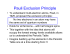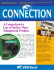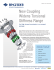# 5.3 Spin-Spin Splitting J-Coupling

## Transcription

5.3 Spin-Spin Splitting J-Coupling
```© Copyright Hans J. Reich 2010
University of Wisconsin
5.3 Spin-Spin Splitting J-Coupling
H
Hα
C
→
→
The actual local magnetic field encountered by a nucleus is affected by neighboring magnetic nuclei (spin-spin
splitting) as a result of perturbation of the electron distribution. The principal magnetic nuclei are other protons, the
100% abundant spin ½ nuclei 19F and ~31P, and some spin 1 or greater (quadrupolar) nuclei such as 14N, 2H, 11B,
and 12B. Although Br, Cl, and I all have isotopes with spin >½, coupling is not seen because of relaxation effects.
This will be discussed in more detail in Section 7.
H
C
Hβ
C
Note: vertical scale of the couplings is grossly exaggerated
C
s
s
E
d
d
t
s
d
dd
dd
Two equal couplings.
t
q
Two different couplings.
Sign of Coupling Constants
Coupling constants can be either positive or negative. These are defined as shown below:
JAX < 0
JAX > 0
A=β
X=α
X=β
X=α
X=β
Coupling constants are positive if the spin state in which A and X have
opposite spins (αβ) is lower in energy than the one in which they have
the same spin (ββ).
The signs of couplings shows some consistency.
• 1JC-H and most other one-bond couplings are positive.
• 2JH-H in sp3 CH2 groups are almost all negative, some others are positive.
A=α
X=β
X=α
X=β
X=β
X=α
X=β
X=α
X=α
• 3JH-H is always positive.
A-signal
d
d
For first order patterns the signs of the couplings have no effect on the
appearance of the spectrum, and so cannot be determined by
observation. However, decoupling experiments (spin tickling) can
provide the relative signs. For second-order patterns (e.g. ABX or
AA'BB'), the relative signs of coupling constants often have dramatic
effects on the appearance of the spectrum, and relative signs can be
determined by proper analysis of the multiplets.
5-HMR-3.1
Two Different Couplings to one Proton
Consider the NMR spectrum of 3,4-dichlorobenzoyl chloride below.
R-19M C7H3Cl3O
270 MHz 1H NMR Spectrum (CDCl3)
Cl
O
Cl
40 30 20 10 0
8.2
9
8
8.1
7
6
8.0
7.9
ppm
5
Cl
Hz
7.8
4
7.7
3
7.6
7.5
2
1
0
ppm
The proton-proton couplings in benzene are typically 7-9 Hz for Jortho, 2-3 Hz for Jmeta and <1 Hz for Jpara. The
substitution pattern can be derived from examination of each of the three aromatic protons. For example, the
doublet at δ 8.2 with J = 2.5 Hz is interpreted as follows: this proton has no protons ortho to it, and only one proton
meta to it. Structure A summarizes the structural information. For the doublet of doublets at δ 7.95 (J = 8.5, 2.3
Hz), formed by coupling of one proton to both an ortho and a meta proton, the two structures B and C are possible.
The doublet at δ 7.6 (J = 8.5 Hz) defines the substitution pattern of structure D. In each case the position marked
by ? is undefined since the para coupling is usually too small to resolve.
X
X
H
H
δ 8.20
?
X
H
X
H
δ 7.95
H
δ 7.95
H
?
H
X
X
H
A
B
C
J ca 2 Hz
one meta H only
J ca 2 and 8 Hz
one ortho and one meta H
5-HMR-3.2
X
X
H
?
?
H
X
δ 7.61
X
D
J = 8 Hz
one ortho H
A slightly more complicated case is 1,1,2-trichloropropane. A simulated spectrum is shown below.
WINDNMR Simulated Spectrum of 1,1,2-Trichloropropane
Coupling constants (in Hz)
2
3
4
J(1:y)
3.80 0.00 0.00
J(2:y)
6.60 6.60
J(3:y)
0.00
J(4:y)
Chemical Shift (in ppm)
δ(1) = 5.850
δ(2) = 4.300
δ(3) = 1.620
δ(4) = 1.620
δ(5) = 1.620
5
0.00
6.60
0.00
0.00
Cl Cl H
H C C C H
Cl H H
8
7
6
5
4
3
2
1
0
ppm
The C-2 proton is coupled to one proton at C-1 and three protons of the methyl group at C-3. Naively, one might
expect a pentet (p), as shown in the left spectrum below. Although pentets are, in fact, often observed in such
situations, this occurs only if J1-2 and J2-3 are identical. When they are not (as is actually the case in this example),
then we get a quartet of doublets (qd). It is customary to quote the larger coupling first (q) and then the smaller
coupling (d). A proper text description of the multiplet is: δ 4.30, 1H, qd, J = 6.6, 3.8 Hz.
Cl Cl
6.6 Hz
Cl C C CH3
H1 H2
Hypothetical
J12 = 6.6 Hz
J23 = 6.6 Hz
4.5
3.8 Hz
Actual
Hypothetical
4.0
J12 = 5.0 Hz
J12 = 3.8 Hz
J23 = 6.6 Hz
J23 = 6.6 Hz
4.5
4.0
Exercise: what would a dq, J = 6.6, 3.8 Hz look like?
5-HMR-3.3
4.5
4.0
First Order Coupling Rules
1. Nuclei must be chemical shift nonequivalent to show obvious coupling to each other. Thus the protons of
CH2Cl2, Si(CH3)4, Cl-CH2-CH2-Cl, H2C=CH2 and benzene are all singlets. Equivalent protons are still coupled to
each other, but the spectra do not show it.
2. J coupling is mutual, i.e. JAB = JBA always. Thus there is never just one nucleus which shows J splitting there must be two, and they must have the same splitting constant J. However, both nuclei need not be protons fluorine (19F) and phosphorus ( 31P) are two other common nuclei that have spin ½ and 100% abundance, so they
will couple to all nearby protons. If these nuclei are present in a molecule, there are likely to be splittings which are
present in only one proton multiplet (i.e. not shared by two multiplets).
3. Two closely spaced lines can be either chemically shifted or coupled. It is not always possible to distinguish J
from δ by the appearance of the spectrum (see Item 4 below). For tough cases (e.g. two closely spaced singlets in
the methyl region) there are several posibilities:
decouple the spectrum
obtain it at a different field strength (measured in Hz, coupling constants are field independent, chemical shifts
are proportional to the magnetic field)
measure the spectrum a different solvent (chemical shifts are usually more solvent dependent than coupling
constants, benzene and chloroform are a good pair of solvents).
For multiplets with more than two lines, areas, intensities, symmetry of the pattern and spacing of the lines
generally make it easy to distinguish chemical shift from coupling.
For a simple example see the spectrum of 3-acetoxy-2-butanone below. Here it is pretty easy to identify one of
the doublets as the 4-methyl group, the other "doublet" (with a separation of 9 Hz, which could easily be a coupling)
actually corresponds to the two CH3C(=O) groups.
300 MHz 1H NMR in CDCl3
Source: Aldrich Spectra Viewer/Reich
O
30
20
10
0
Hz
O
5.88
O
2.91
5.15 5.10 5.05 5.00
1.00
2.20 2.15 2.10
5
4
3
1.40
2
1
ppm
4. Chemical shifts are usually reported in δ (units: ppm) so that the numeric values will not depend on the
spectrometer frequency (field-independent units), coupling constants are always reported in Hz (cycles per
second). Chemical shifts are caused by the magnetic field, couplings are field-independent, the coupling is
inherent in the magnetic properties of the molecule. However, all calculations on NMR spectra are done using Hz
(or, more precisely, in radians per sec).
5-HMR-3.4
0
H
5. Protons two (2J, geminal) or three bonds ( 3J, vicinal) apart are usually coupled to each other, more remote
protons (4J, 5J) may be if geometry is right, or if π-systems (multiple bonds) intervene. Long range couplings ( 4J or
greater) are usually small, typically <0.5 Hz, but up to 3 Hz in some cases where there are intervening π bonds.
H
2
J = 2-15 Hz
Typical: -12 Hz
C
H
geminal
H
H
Note
small
0-3 Hz
C
H
C
3
C
H
vicinal
J = 2-20 Hz
Typical: 7 Hz
Occasionally 0!
H
4
C
C
J = 0-3 Hz
Usually 0
H
long-range
6. Multiplicity for first order patterns follows the "doubling rule". If all couplings to a particular proton are the
same there will be 2nI+1 lines, where I is the spin and n is the number of neighboring nuclei (n + 1 for 1H I = 1/2).
The intensities will follow Pascal's triangle.
n=0
n=1
n=2
n=3
n=4
n=5
n=6
1
1 1
1 2 1
1 3 3 1
1 4 6 4 1
1 5 10 10 5 1
1 6 15 20 15 6 1
Intensities
triplet n = 2
quartet n = 3
pentet n = 4
sextet n = 5
nonet n = 8
7. If all couplings are different, then the number of peaks is 2n for 1H, and the intensities are 1:1:1: . . .. Thus a
proton coupled to two others by different couplings gives a dd (doublet of doublets, see Figure). This pattern is
never called a quartet. As the number of couplings gets larger, accidental superpositions of lines will sometimes
occur, so that the 1:1:1... intensity ratio no longer applies. The intensities are also often distorted by leaning effects,
as seen in several examples below.
dd n = 2
ddd n = 3
ddd n = 3
dddd n = 4
ddddd n = 5
8. More typically, some of the couplings are the same, others different, so get a variety of patterns. In
favorable cases, these patterns can be analyzed and all couplings extracted. The number and size of couplings
(J-values) provide important structural information.
5-HMR-3.5
Second Order Effects
1. Protons or groups of protons form simple multiplets only if the chemical shift differences between the protons
(Δν) are large compared to the coupling constants between them (J). If Δν /J (all in Hz) is <5 then second order
effects appear (see 5.8) which complicate the analysis:
(a) A universally observed effect is that the intensities of the lines no longer follow simple integer ratios - the
multiplets "lean" towards each other: the lines away from the chemical shift of the other proton (outer lines) become
smaller and lines closer (inner lines) become larger (see the triplets below). The leaning becomes more pronounced
as the chemical shift difference between the coupled multiplets becomes smaller.
C3H7BrO
300 MHz 1H NMR spectrum in CDCl3
Source: Aldrich Spectra Viewer/Reich
3.8
"leaning"
3.7
8
Br
O
"leaning"
3.6
3.5
7
3.4
6
5
ppm
4
3
2
1
0
(b) Additional lines over that predicted by simple coupling rules appear. A nice example is provided by the
compound below. For the BrCH 2CH2O group the two methylenes at δ 3.48 and δ 3.81 have a relatively large
chemical shift separation, and they form recognizable triplets, although with a little leaning. For the MeOCH 2CH2O
group the chemical shift between the CH 2 groups is small, and the signals are a complicated multiplet with only a
vague resemblance to a triplet. There is likely an additional complication from variability in the size of the two
different vicinal couplings in the two patterns (see section 5-HMR-15 for more on this).
C5H11BrO2
300 MHz 1H NMR spectrum in CDCl3
Source: Aldrich Spectra Viewer/Reich
O
3.8
8
3.7
7
Br
O
3.6
3.5
6
3.4
5
ppm
4
5-HMR-3.6
3
2
1
0
(c) Coupling constants and chemical shifts can no longer be easily determined from the spectrum. The sign of the
coupling constant is significant. "Virtual coupling" effects (apparent coupling to protons that are actually not coupled)
may appear (see Section 5.16).
2. For a given molecule Δν /J increases as the magnetic field increases, hence spectra are usually simpler at
higher field (better dispersion).
3. Second order effects will appear even if Δδ /J is large when groups of magnetically non-equivalent protons
with identical chemical shifts are coupled to each other (see Section 5.8). Thus Me 3Si-CH2-CH2-Cl (an AA'XX'
system, see Section 5.15) is not just two triplets. These patterns do not get simpler at higher field strengths.
These effects will be dealt with in some detail in subsequent sections on the various coupling patterns. 01/39
5-HMR-3.7
5.3.7 Rules for Analyzing First Order Multiplets
A first order multiplet can be expected when both of the following criteria are met:
First, the chemical shift of the observed proton must be far away from any of the protons it is coupled to (far
away means Δν >> J). In practice, multiplets can be treated in a first order fashion if Δν > 3J, although the
substantial leaning distortions can complicate analysis. The leaning will have almost completely disappeared by the
time Δν = 10J.
Second, if more than one proton is coupled to the observed one, then these protons must not be "strongly
coupled." In othere words, if they are coupled to each other and very close in chemical shift then the observed
proton multiplet will not yield true coupling constants on analysis, even though it looks first order. See the section on
Virtual Coupling.
Structure of First Order Multiplets. The fundamental rule governing multiplet intensities for spin 1/2 nuclei with
all couplings identical is Pascal's triangle (n = number of equivalent couplings).
n
2n
0
1
2
3
4
5
6
7
8
1
2
4
8
16
32
64
128
256
Multiplet Intensities
1
1 1
1 2 1
1 3 3 1
Pentet 1 4 6 4 1
Sextet 1 5 10 10 5 1
Septet 1 6 15 20 15 6 1
Octet 1 7 21 35 35 21 7 1
Nonet 1 8 28 56 70 56 28 8 1
A first order multiplet consists of the product (not the sum) of several such multiplets. In other words, a single line
will first be split into one of the symmetrical multiplets (1:1 d, 1:2:1 t, 1:3:3:1 q, etc), then each line of this multiplet
will be again split into d, t, q, or higher multiplet.
5-HMR-3.8
Recognizing a First Order Multiplet.
1. All truly first order multiplets are centrosymmetric - there is a mirror plane in the middle (in real spectra, this is
usually not strictly true because of leaning and other distortions). However, the reverse is not true: not all
symmetrical multiplets are first order.
2. If the small outermost peaks are assigned intensity 1, then all other peaks must be an integral multiple
intensity of this one (1x, 2x, 3x, 4x in height), and the total intensity of all peaks must be a power of 2 (2, 4, 8, 16, 32,
etc). The intensity of each of the two outermost lines is 1/2 n of the total multiplet intensity, where n is the number of
protons which are coupled with the proton signal being analyzed. There can be no lines smaller than the outermost
one. Note, however, that if n is large, the outermost peaks may not be distinguishable from noise. Intensity
assignments and determination of n cannot be easily made for such multiplets
3 3
6 6
2
2
2
1
1 1 1 1 11
3 33 3
11
11 1
2
1 1
2
1 1
Σ=8
Σ = 16
Σ = 32
Coupled to 3 other protons
23 = 8
Coupled to 4 other protons
24 = 16
Coupled to 5 other protons
25 = 32
3. There is a strict regularity of spacing in a first order multiplet: if you have correctly identified a coupling
constant J, then every peak in the multiplet must have a partner J Hz away to the left or to the right of it.
3
3
2
This separation is
always a true coupling
2
1
1 1
1 1
1
This is also a coupling
This is not a coupling
This is not a coupling
4. Most first order multiplets integrate to a single proton, a few may be 2 or 3 protons in area. It is rare to have
more than 3 protons, unless there is symmetry in the molecule (e.g., (CH 3)2CH- gives a 6-proton doublet for the
methyl groups). Thus a 4-proton symmetrical multiplet is usually not a first-order pattern (it is more likely to be the
very common AA'BB' pattern).
5. The symmetry and intensities of an otherwise first-order multiplet can be distorted by leaning effects (see
Section 5-HMR-9). Many such multiplets can still be correctly analyzed by first-order techniques, but you have to
mentally correct for the intensity distortions. However, the coupling constants extracted may not be perfectly
accurate.
5-HMR-3.9
Analyzing a First Order Multiplet. First order multiplets are analyzed by constructing a reverse coupling tree, by
"removing" each of the couplings in turn, starting with the smallest.
1. "Take out" the smallest couplings first. The separation between the two lines at the edge of the multiplet is
the smallest coupling. Each time you remove a coupling you generate a new, simpler multiplet, which can then be
analyzed in turn. Remember that each line of the multiplet participates in each coupling.
2. Watch line intensities (i.e., peak areas or peak heights) carefully--when you "take out" a coupling, the
intensities of the newly created lines should be appropriate (i.e., each time you "take out" a coupling, also "take out"
the proper intensity). When a coupling has been taken out completely, all intensity should be accounted for. Keep
track of your analysis by using a "coupling tree".
3. The couplings may be removed one at a time as doublets, or as triplets, quartets and higher multiplets. The
intensity ratio of the first two lines signals the number of protons involved in the coupling: 1:1 means there is only
one proton, 1:2 means that there is a triplet splitting (2 protons), etc. Be especially careful to keep track of intensities
when you "take out" triplets (1:2:1) or quartets (1:3:3:1). Each time you completely remove a coupling you generate
a new multiplet which follows first order rules, and can be analyzed in turn.
When you have finished your analysis, all peaks in the multiplet must be accounted for. You can check the
analysis as follows: the separation of the two outermost peaks of the multiplet is the sum of all the J's (i.e., for a dt, J
= 8, 3 Hz the outermost lines are separated by 8 + 3 + 3 = 14 Hz).
Reporting a First Order Multiplet. Multiplets are reported starting with the largest coupling, and the symbols
must be in the order of the reported numbers: δ 2.10, 1H, qt, J = 10, 6 Hz means: a single proton q of 10 Hz, t of 6 Hz
with a chemical shifts of 2.10 ppm.
Quartets. Keep clear in your mind the distinction between a simple q (one proton equally coupled to 3 others,
with an intensity 1:3:3:1), an ABq (2 protons coupled to each other, see Sect 5-HMR-9), and the quartet formed by
coupling with a spin 3/2 nucleus (e.g., 7Li, intensity 1:1:1:1, see Sect 7-MULTI-2.4). Only the first of these should be
referred to by just a "q" symbol. The early NMR literature (and even modern novices) sometimes call doublets of
doublets "quartets" (there are four lines, after all).
quartet
AB quartet
1:1:1:1 quartet
5-HMR-3.10
doublet of doublets
Chem 605
Practice Multiplets
Reich
J
J
50
40
30
20
Hz
5-HMR-3.11
10
0
Σ = 8 (3 couplings present)
First Order Analysis
1
1
11 11
1
1
The separation between the first and second lines is the smallest
coupling in the multiplet (J1). They are in an intensity ratio of 1:1, so
that coupling appears only once (i.e., it is a doublet splitting).
"Remove" the first coupling. Keep track of line intensities, and draw
a child multiplet with positions at the center of each doublet. Each
unit of line intensity can only be used once, and all line intensity
must be accounted for
J1
Repeat the process - the first and second lines now represent the
next largest coupling. Remove this in turn, and repeat the process
until you get a singlet
J2
J3
This is ddd, J = 12, 10, 4 Hz
Σ = 16 (4 couplings present)
3
Here again the separation of the first two lines is the smallest
coupling in the multiplet (J1). However, they are in an intensity ratio
of 1:2, so this coupling appears twice (i.e., it is a triplet splitting).
3
2
1
2
1 1
1 1
1
"Remove" the triplet coupling. Keep track of line intensities, and
draw a child multiplet with positions at the center of each triplet.
Note that the central two lines of intensity 3 are the sum of two lines
- one of intensity 1 and the other intensity 2.
J1
This is a ddt, J = 16, 10, 6 Hz
J2
J3
5-HMR-3.12
Simple Multiplets
Assign the protons where structure and chemical shift scale are given
Note the leaning, indicating that the coupled partner is close by.
Doublet of doublets (dd)
Triplet of doublets or doublet of triplets (dt, td)
O
O
O
O
Ph
O
O
H
Cl
O
2.45 2.40
ppm
3.05 3.00
ppm
3.00 2.95
ppm
2.70 2.65 2.60
ppm
Doublet of doublet of doublets (ddd)
O
40 30 20 10 0
O
Hz
O
2.55 2.50 2.45
ppm
5.85 5.80
ppm
Doublet of quartets (dq)
The multiplet below integrates to two protons.
Using the chemical shift (δ 5.8) and coupling
identify the structural fragment.
O
O
OMe
7.05 7.00 6.95 6.90
ppm
OMe
5.90 5.85 5.80
ppm
5-HMR-3.13
5.95 5.90 5.85 5.80 5.75 5.70 5.65
ppm
Symmetrical Multiplets which are NOT First Order
Only ONE of the multiplets below is first order
Some criteria to use:
Pattern must be centrosymmetric (true of all of these)
Intensity of lines - patterns must be repeated, especially examine outer lines
Be wary if #H > 1, especially if 4H
Consider size of possible couplings
(a)
(b)
50
40
30
20
10
0
Hz
(c)
(d)
(e)
(f)
5-HMR-3.14
5-HMR-3.15 Measurement of Coupling Constants
The accurate measurement of J coupling constants requires that the multiplets be correctly analyzed. In the
following pages are described techniques for performing such analyses. The procedures are summarized below.
For first order multiplets a simple "tree" analysis as described in Section 5-HMR-3.9 can directly yield coupling
constants within the accuracy of the digital resolution of the spectrum. This includes AB spectra, where JAB can be
measured directly. See section 5-HMR-7 for a description of the ABC.. nomenclature for spin systems.
For AB2 spectra both the coupling constant JAB and the chemical shifts can be obtained by simple arithmetic
manipulations, provided that line assignments can be made correctly. For ABX spectra JAB is accurately
measureable by inspection. An approximate analysis, which treats the peaks as AMX, will give values for JAX and
JBX that will be in error by varying amounts, depending on the relative size of νAB, the relative size of JAX and JBX
and the size of JAB (the smaller νAB the larger the error). To get accurate values for the JAX and JBX coupling
constants a proper ABX analysis as described in Section 5-HMR-12 is required.
For many simple compounds the symmetry is such that protons are homotopic or enantiotopic, and no coupling
constants can be measured directly (e.g., the 2J coupling in methane or dichloromethane; the ortho, meta, and para
couplings in benzene; the cis, trans and gem couplings in ethylene, etc). For such compounds the following
techniques are used to measure JHH:
Analysis of Complex Spin Systems. In molecules where the chemical shift-equivalent protons are of the AA'
type (part of an AA'XX', AA'X3X3' or similar system), complete analysis of the spectrum sytem can, in favorable
circumstances, give the value of JAA'. An example is 1,3-butadiene, an AA'BB'CC' system in which all protons are
compled to all other ones. Analysis of the complex NMR spectrum gave, among numerous other couplings, values
for the following couplings between chemical shift equivalent nuclei: 3JAA', 5JBB' and 5JCC' (Hobgood, R. T., Jr.;
Goldstein, J. H. J. Mol. Spectr. 1964, 12, 76).
νA
νC νB
HA'
HC
HB'
60 MHz
Solv: cyclohexane
HB
HC'
HA
3
3
JAB = 10.17
4
5
JBB' = 1.30
4
JAB' = -0.86
2
5
JCC' = 0.69
3
JAA' = 10.41
JAC = 17.05
JAC' = -0.83
JBC = 1.74
5
JBC' = 0.60
6.6
6.4
6.2
6.0
5.4
5.2
5.0
Isotopic Substitution. Replacing one of the protons by deuterium (or even tritium) breaks the symmetry of the
coupled system and allows measurement of JHD (or JHT). The value of JHH can then be calculated from the
gyromagnetic ratios. In the example below, the 60 MHz NMR spectrum of a mixture of undeuterated (s),
monodeuterated (1:1:1 triplet, the spin of D is 1, see Sect. 7-MULTI-2.1) and dideuterated (1:2:3:2:1 quintet)
acetonitrile is shown. Note the isotopic shifts. (Grant, D. M.; Barfield, M. JACS 1961, 83, 4727)
CH3CN
JHH
JHD
JHH
=
γH
γD
=
6.488
6.488 × JHD
JHD (CH2D-CN) = 2.58 Hz
CDH2CN
CD2HCN
120
=
JHH (CH3-CN) = 16.75 Hz
115
Hz
5-HMR-3.15
4.8
Analysis of 13C Satellite Spectra. Vicinal couplings between homotopic or enantiotopic protons 3JHH can often
be obtained by analysis of the 13C satellites. The 1H NMR signal for the vinyl protons of dimethyl maleate is a
singlet. However, the 13C satellites are doublets, with a splitting that is equal to 3JHH. In effect, the A2 spin system of
the 12C isotopomer has become an ABX pattern in the mono- 13C labelled compound, where X is the 13C nucleus,
and A and B are the two vinyl protons, one on 13C and the other on 12C.
For systems of the X-CH2-CH2-X type, the mono-13C isotopomer is an AA'BB'X pattern, which can be solved to
obtain JAA' (= JBB') as well as JAB and JAB'.
Spinning
side bands
C6H8O4
CDCl3
200 MHz
O
13
C Satellite
13
C Satellite
H
OMe
1
JHC
X
3
OMe
H
JHH
O
O
1300
1250
1200
H 13
C
1150
OMe
12
H
C
OMe
O
8
7
6
5
4
2.2% of the molecules have
at one of the vinyl carbons
3
2
13
C
1
0
ppm
C3H6O3
O
H
O
H
The BB' protons
are hidden under
the central peak
3.97
12
C
O
O
CDCl3, 300 MHz
W. S. Goldenberg
O
O
13
HA
1
JHC
H
H
AA'BB'X
2.00
13
C
O
5.2
9
5.0
4.8
8
HA' HB'
1
JHC
O
4.0
4.6
7
6
C 12C
5
ppm
5-HMR-3.16
4
3
3.8
2
3.6
1
0
HB
Below is an example of the measurement of a 4JHH in a symmetric tricyclic system using the 13C satellite method
Masamune, S. J. Am. Chem. Soc. 1964, 86, 735
Ph
Ph
4
H J = 14 Hz
H
O
1
JHC = 190.1 Hz
4
JHH = 14 Hz
2.88 (190)
8
7
6
5
4
ppm
5-HMR-3.17
3
2
1
0
```

### Fernco Flexible Coupling Installation - Fernco### GEISLINGER COUPLING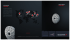### Spin-Spin Splitting in Alkenes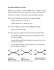### Chpt 8